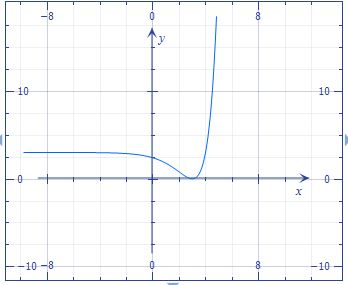# Draw the following function and find its critical points, if any: f(x) = 3(x-4)e^{x-3}+3

## Question:

Draw the following function and find its critical points, if any: {eq}f(x) = 3(x-4)e^{x-3}+3 {/eq}

## Critical Points:

We can find the critical points of a function where the curve changes from increasing to decreasing or from decreasing to increasing. We can also find the critical points by equating the first derivative to zero or when it does not exist.

We have the function

{eq}f(x) = 3(x-4)e^{x-3}+3 {/eq}

Graph the function:Equating the first derivative to zero, we find the critical points.

Differentiating the function

{eq}f'(x)=3\,{{\rm e}^{x-3}}+3\, \left( x-4 \right) {{\rm e}^{x-3}} \\ {/eq}

{eq}f'(x)=0 {/eq} when {eq}x=3 \\ {/eq}

Therefore, Critical point at:

{eq}(3, 0) {/eq}